# Recursion or Recursive Function in C

In this article, we will learn about recursion in C with an example. That is, you will get a brief explanation of the working principle of a recursive function in C.

## What are recursive functions?

When a function calls itself during its definition or execution, it is known as a recursive function. For example, a function named printFiveTime() calls itself to print anything, say my name, five times, as shown here in the following program:

```#include <stdio.h>
#include<conio.h>
int printFiveTime(int);
int main()
{
printFiveTime(5);
getch();
return 0;
}
int printFiveTime(int t)
{
if (t == 0)
return 0;
else
{
printf("codescracker\n");
printFiveTime(t - 1);
}
}```

This program was written in the Code::Blocks IDE. Here is the sample run: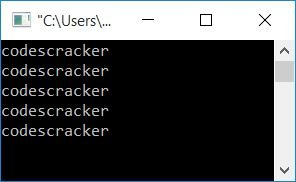As you can see in the preceding program, the function "printFiveTime()" calls itself within the function. In other words, "printFiveTime()" is called from within the function definition. As a result, the above function "printFiveTime()" is a recursive function.

## How does a recursive function get executed?

Memory will be allocated inside the RAM to execute a function in the application (here, memory is referred to as stack memory). That is, to execute a function (or method, or block), some amount of memory will be allocated inside the stack memory, which is called a frame. For each function or method execution, a specific amount of memory is allocated.

As a result, the operating system calls the "main()" function first when we run the preceding program. Some memory is set aside to allow the "main()" function to run. So the "main" frame will be created here. As a result, enough memory will be allocated to execute all three statements given inside the above program's main function, as shown in the snapshot below: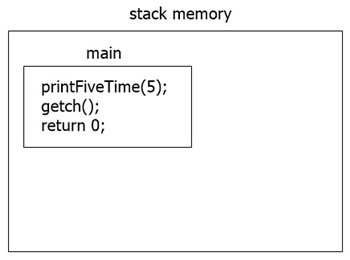As you can clearly see, the frame for the main() function gets created inside the stack memory. Here in the first statement, we are calling the printFiveTime()  function, so for this function, another frame will be created like this: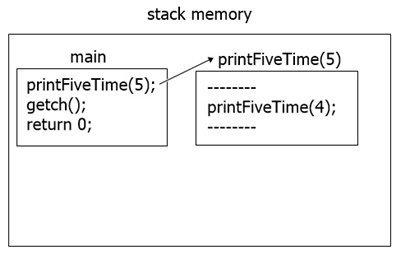In the above figure, ------- represents other statements of the function printFiveTime(). Now let's get back to the code. First, we are passing 5 to the function printFiveTime(). As a result, 5 is passed to the function definition on the first run. Therefore, the function gets executed like this:

```printf("codescracker\n");
printFiveTime(5-1);```

While passing 5 to the function printFiveTime() at first, the variable t holds 5. Program flow goes inside the function definition part, as 5 is not equal to 0, therefore program flow goes to the else part, and the above two statements get executed.

As t-1 or 5-1 gives 4, we have just passed 4 to the printFiveTime() function. Now, for this function, another frame gets created. The frame continues creating until the value of t becomes 0, as shown in the image given here: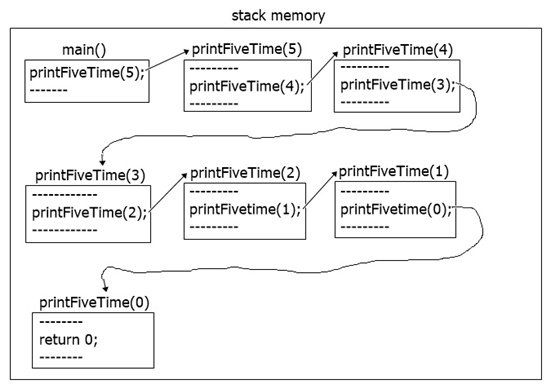In all the frames except printFiveTime(0), the else block gets executed, but in the last frame, printFiveTime(0), the if block gets executed. In the else block, the following statement:

`return 0;`

gets executed. So here, once all the function space gets clearly defined with its value, all the frames get deleted. In a recursive function, control goes in the forward direction until and unless all the frame's statements get clearly executed. And in whatever order the control goes (in a forward direction), in the same order the control should come back, and where it starts the execution, only there should it complete the execution.

So here, when the value of t becomes 0, the program flow comes back to where it started the execution, and all the statements get executed. The control then goes to the main function, and the last two statements get executed, which are:

```getch();
return 0;```

To clarify the above statement (about the flow of forward and backward directions in recursive functions), you can print the value of t along with codescracker as shown in the following program:

```#include<stdio.h>
#include<conio.h>
int printFiveTime(int);
int main()
{
printFiveTime(5);
getch();
return 0;
}
int printFiveTime(int t)
{
if(t==0)
return 0;
else
{
printf("%d. codescracker\n", t);
printFiveTime(t-1);
}
}```

The snapshot given below shows the sample run: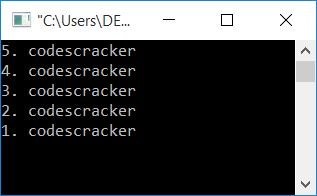C Online Test

« Previous Tutorial Next Tutorial »